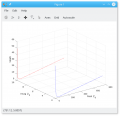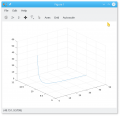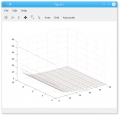Antenna impedance - question about types of impedances

PsySc0rpi0n

Joined Mar 4, 2014
1,446
Hello again.

I'm at this point working on my final year project and I have some questions that probably I should not have, but I do.

I'm following a book to help me with formulas and to better understand the concepts I need to understand. This book is "Antenna Theory. Analysis and Design" from Constantine, A. Balanis.

At this point I'm bringing back to my memory concepts such as reflection coefficient and voltage standing wave ratio that I need for my project.

So, at a starting point, I was asked to write some code in MatLab/Octave to try to better understand the impact on VSWR inherent to changing the Transmission Line impedance, Antenna impedance and Antenna impedance types (such as inductive and capacitive impedance).

So, from the book the reflection coefficient is given by:

$\displaystyle{\Gamma = \frac{Z_{in}-Z_{0}}{Z_{in}+Z_{0}}}$

But then I'm left thinking how can I include a non purely resistive load in this formula. I mean, if the load has an inductor or a capacitor, how do I evaluate the reflection coefficient?

KL7AJ

Joined Nov 4, 2008
2,208
Hello again.

I'm at this point working on my final year project and I have some questions that probably I should not have, but I do.

I'm following a book to help me with formulas and to better understand the concepts I need to understand. This book is "Antenna Theory. Analysis and Design" from Constantine, A. Balanis.

At this point I'm bringing back to my memory concepts such as reflection coefficient and voltage standing wave ratio that I need for my project.

So, at a starting point, I was asked to write some code in MatLab/Octave to try to better understand the impact on VSWR inherent to changing the Transmission Line impedance, Antenna impedance and Antenna impedance types (such as inductive and capacitive impedance).

So, from the book the reflection coefficient is given by:

$\displaystyle{\Gamma = \frac{Z_{in}-Z_{0}}{Z_{in}+Z_{0}}}$

But then I'm left thinking how can I include a non purely resistive load in this formula. I mean, if the load has an inductor or a capacitor, how do I evaluate the reflection coefficient?
Well, unless you LIKE calculating complex hyperbolic trig functions, I suggest using the Smith Chart.

Papabravo

Joined Feb 24, 2006
12,301
Complex numbers require more effort to compute manually than real numbers in such formulas. You substitute, then plug and chug. Matlab and Octave can help in this regard.

PsySc0rpi0n

Joined Mar 4, 2014
1,446
Yeah, actually I'm using Octave to try to plot this behaviour!

So, from what I've seen from simulation in LTSpice, for both Short Circuit open circuit as a load I expect very high values of VSWR, infinity will consider it! And for 50Ω load, I expect a VSWR of 1. So, globally I was expecting a curve with a parabolic shape!

So what I'm doing in Octave is to define an L, a C, an R, a frequency and a Transmission Line impedance! Then I compute the reflection coefficient and finally I compute the VSWR value and try to plot VSWR wrt Z_0.

Being:
L the load inductance value
C the load capacitance value
R the load resistance value
X_L the load inductive reactance
X_C the load capacitive reactance
Z_tl the Transmission Line impedance
Z_0 the load impedance
refcoeff the reflection coefficient
VSWR the voltage standing wave ratio

So the code I did was the following:

Code:
clear all;
clc;
close all;

freq = 1e6;
L = [1e-9:1e-9:10e-6];
C = 0;
R = 51;
Zin = 50;

if (C != 0 && L != 0)
X_L = j*2*pi*freq*L;
X_C = 1/(j*2*pi*freq*C);
elseif (!C)
X_L = j*2*pi*freq*L;
X_C = 0;
elseif (!L)
X_L = 0;
X_C = 1/(j*2*pi*freq*C);
endif
Z_0 = R + j*(X_L + X_C);
refcoeff = (Zin - Z_0) ./ (Zin + Z_0);
VSWR = (1 + abs(refcoeff)) ./ (1 - abs(refcoeff));

plot(VSWR, Z_0, "markersize", 10);
But I'm going to change this yet a bit! I want to compare the curves of VSWR when we have a purely resistive antenna, an antenna with inductive load (a couple of values) and an antenna with a capacitive load (also a couple of values).

PsySc0rpi0n

Joined Mar 4, 2014
1,446
Ok, I'm now with some good results for a simple plot of 2 values for 2 different iductances but when I try to make thr code a bit more dynamic, namely using matrices to hold several values of inductances, plot form/shape changes quite a bit and I can't understand why.

I have tested the simpler code with 2 values for L and the plot looks pretty good.
Then I tried the more dynamic code and the plot is quite different. Can you help me understand why it is so different?

Simple code with 2 L values
Code:
clear all;
clc;
close all;

freq = 1e6;
modZL=2;
L=modZL/(2*pi*freq);
C = 0;
R = [0:1:750];
Zin = 50;

if (C != 0 && L != 0)
X_L = j*2*pi*freq*L;
X_C = 1/(j*2*pi*freq*C);
elseif (!C)
X_L = j*2*pi*freq*L;
X_C = 0;
elseif (!L)
X_L = 0;
X_C = 1/(j*2*pi*freq*C);
endif

Z_0 = R + (X_L + X_C);

refcoeff = (Zin - Z_0) ./ (Zin + Z_0);
VSWR = (1 + abs(refcoeff)) ./ (1 - abs(refcoeff));

plot3(real(Z_0),imag(Z_0),VSWR,'b');
grid on;
xlabel("Real Z_0");
zlabel("VSWR");
ylabel("Imag Z_0");
hold on;

modZL=10;
L=modZL/(2*pi*freq);

if (C != 0 && L != 0)
X_L = j*2*pi*freq*L;
X_C = 1/(j*2*pi*freq*C);
elseif (!C)
X_L = j*2*pi*freq*L;
X_C = 0;
elseif (!L)
X_L = 0;
X_C = 1/(j*2*pi*freq*C);
endif

Z_0 = R + (X_L + X_C);

plot3(real(Z_0),imag(Z_0),VSWR,'r');

This plot is the simple code with 2 values of L. This plot has 2 lines and the displayed the way they are.This next code is the dynamic one and if I use only 1 value for L, the plot is ok. If I use more than one value, plot shape changes.
Code:
clear all;
clc;
close all;

freq = str2num(input("Enter frequency:\n", "Signal Frequency\n"));
numLs = str2num(input("How many inductances to test:\n", "Inductances test\n"));

R = [0:1:50];
Zin = 50;
modXLvals = zeros(1, numLs);
L = zeros(1, numLs);
XL = zeros(1, numLs);
Z_0 = zeros(numLs, numel(R));

for i = 1:numLs
printf("Enter inductance value nº %d\n", i);
modXLvals(i) = input("");
L(i) = modXLvals(i)./(2*pi*freq);
XL(i) = j*2*pi*freq*L(i);
endfor

for i = 1:numLs
for j = 1:numel(R)
Z_0(i,j) = R(j) + XL(i);
endfor
endfor

for i = 1:numLs
for j = 1:numel(R)
refcoeff(i, j) = (Zin - Z_0(i, j)) ./ (Zin + Z_0(i, j));
endfor
endfor

for i = 1:numLs
for j = 1:numel(R)
VSWR = (1 + abs(refcoeff)) ./ (1 - abs(refcoeff));
endfor
endfor

plot3(real(Z_0), imag(Z_0), VSWR)
grid on;

This is the dynamic plot with one value of LAnd this is the plot with 2 values for L which plots an whole bunch of lines and displayed in a different way when using only 1 value for L.
What I expected for this last plot was something very similar (theoretically equal) to the first plot!Can anyone help me figuring out why this is happening?

PsySc0rpi0n

Joined Mar 4, 2014
1,446
So no one can help me with the code?